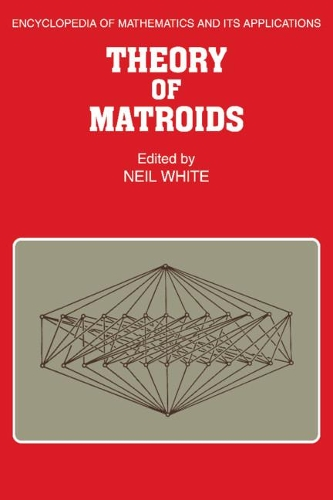•Encyclopedia of Mathematics and its Applications: Theory of Matroids Series Number 26 (Paperback)

(editor)
£58.99
Paperback 340 Pages / Published: 04/12/2008
• Not available

This product is currently unavailable.

The theory of matroids is unique in the extent to which it connects such disparate branches of combinatorial theory and algebra as graph theory, lattice theory, design theory, combinatorial optimization, linear algebra, group theory, ring theory and field theory. Furthermore, matroid theory is alone among mathematical theories because of the number and variety of its equivalent axiom systems. Indeed, matroids are amazingly versatile and the approaches to the subject are varied and numerous. This book is a primer in the basic axioms and constructions of matroids. The contributions by various leaders in the field include chapters on axiom systems, lattices, basis exchange properties, orthogonality, graphs and networks, constructions, maps, semi-modular functions and an appendix on cryptomorphisms. The authors have concentrated on giving a lucid exposition of the individual topics; explanations of theorems are preferred to complete proofs and original work is thoroughly referenced. In addition, exercises are included for each topic.

Publisher: Cambridge University Press
ISBN: 9780521092029
Number of pages: 340
Weight: 500 g
Dimensions: 229 x 152 x 19 mm